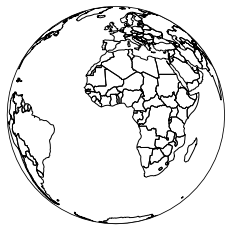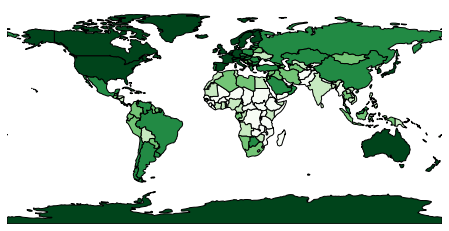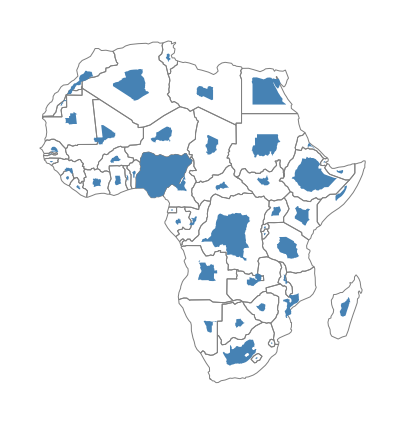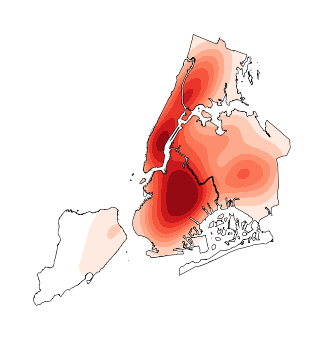# Plotting with Geoplot and GeoPandas¶

Geoplot is a Python library providing a selection of easy-to-use geospatial visualizations. It is built on top of the lower-level CartoPy, covered in a separate section of this tutorial, and is designed to work with GeoPandas input.

This example is a brief tour of the geoplot API. For more details on the library refer to its documentation.

First we’ll load in the data using GeoPandas.

:

import geopandas
import geoplot

geopandas.datasets.get_path('naturalearth_lowres')
)
geoplot.datasets.get_path('nyc_boroughs')
)
geoplot.datasets.get_path('nyc_injurious_collisions')
)


# Plotting with Geoplot¶

We start out by replicating the basic GeoPandas world plot using Geoplot.

:

geoplot.polyplot(world, figsize=(8, 4))

:

<AxesSubplot:>Geoplot can re-project data into any of the map projections provided by CartoPy (see the list here).

:

# use the Orthographic map projection (e.g. a world globe)
ax = geoplot.polyplot(
world, projection=geoplot.crs.Orthographic(), figsize=(8, 4)
)
ax.outline_patch.set_visible(True)

/home/docs/checkouts/readthedocs.org/user_builds/geopandas/conda/v0.11.0/lib/python3.9/site-packages/geoplot/geoplot.py:711: UserWarning: Plot extent lies outside of the Orthographic projection's viewport. Defaulting to global extent.
warnings.warn(
/tmp/ipykernel_3666/975099287.py:5: DeprecationWarning: The outline_patch property is deprecated. Use GeoAxes.spines['geo'] or the default Axes properties instead.
ax.outline_patch.set_visible(True)polyplot is trivial and can only plot the geometries you pass to it. If you want to use color as a visual variable, specify a choropleth. Here we sort GDP per person by country into five buckets by color, using “quantiles” binning from the Mapclassify library.

:

import mapclassify
gpd_per_person = world['gdp_md_est'] / world['pop_est']
scheme = mapclassify.Quantiles(gpd_per_person, k=5)

# Note: this code sample requires geoplot>=0.4.0.
geoplot.choropleth(
world, hue=gpd_per_person, scheme=scheme,
cmap='Greens', figsize=(8, 4)
)

:

<AxesSubplot:>If you want to use size as a visual variable, use a cartogram. Here are population estimates for countries in Africa.

:

africa = world.query('continent == "Africa"')
ax = geoplot.cartogram(
africa, scale='pop_est', limits=(0.2, 1),
edgecolor='None', figsize=(7, 8)
)
geoplot.polyplot(africa, edgecolor='gray', ax=ax)

:

<AxesSubplot:>If we have data in the shape of points in space, we may generate a three-dimensional heatmap on it using kdeplot.

:

ax = geoplot.kdeplot(
projection=geoplot.crs.AlbersEqualArea())
geoplot.polyplot(boroughs, ax=ax, zorder=1)

/home/docs/checkouts/readthedocs.org/user_builds/geopandas/conda/v0.11.0/lib/python3.9/site-packages/seaborn/_decorators.py:36: FutureWarning: Pass the following variable as a keyword arg: y. From version 0.12, the only valid positional argument will be data, and passing other arguments without an explicit keyword will result in an error or misinterpretation.
warnings.warn(
/home/docs/checkouts/readthedocs.org/user_builds/geopandas/conda/v0.11.0/lib/python3.9/site-packages/seaborn/distributions.py:1718: UserWarning: shade_lowest is now deprecated in favor of thresh. Setting thresh=0.05, but please update your code.
warnings.warn(msg, UserWarning)

:

<GeoAxesSubplot:>These are just some of the plots you can make with Geoplot. There are many other possibilities not covered in this brief introduction. For more examples, refer to the Gallery in the Geoplot documentation.